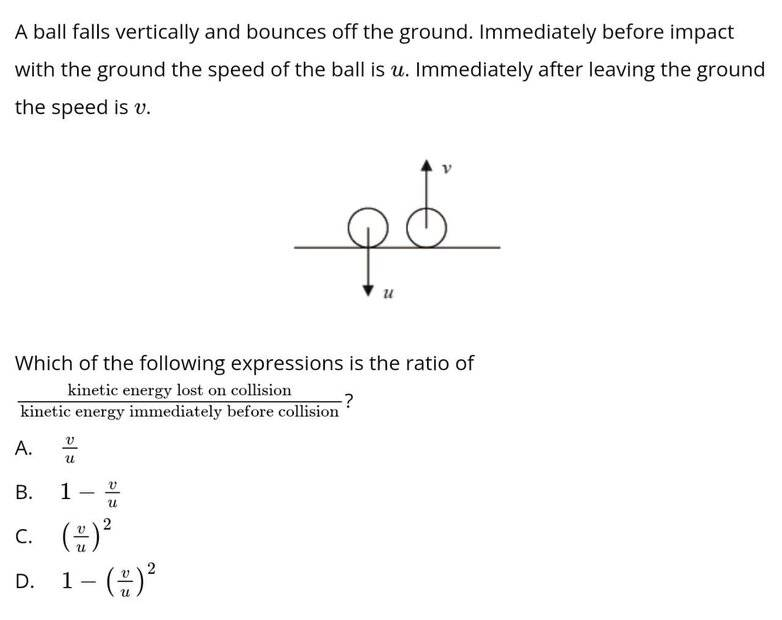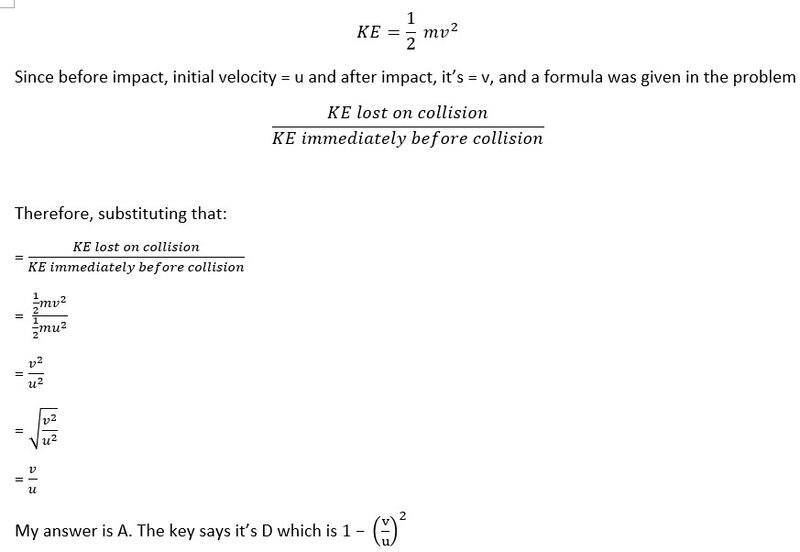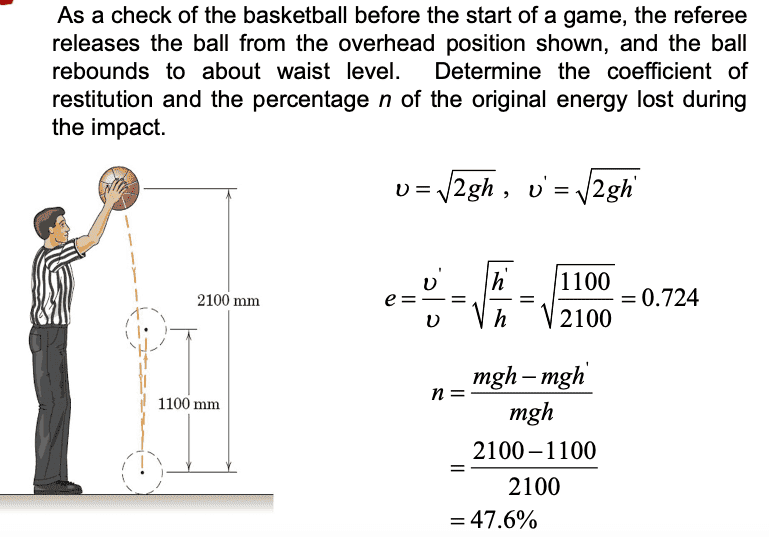# About Kinetic Energy of a ball falling vertically and bouncing up

Iwanttolearnphysics
Homework Statement:
"A ball falls vertically and bounces off the ground. Immediately before impact with the ground, the speed of the ball is u. Immediately after leaving the ground the speed is v. Which of the following expressions is the ratio of (kinetic energy lost on collision/kinetic energy immediately after a collision)?
Relevant Equations:
KE = 1/2mv^2
Hello again. I don't believe there are rules about posting twice in a day. I'm not a student and I answer Physics questions as a hobby, but I've only just started learning, so please help me out. I'm answering IBDP Physics questions.Here's my thinking:
• KE is not a vector quantity, so it's always just written as is.
• Velocity is a vector quantity, so taking into account that u moves downwards, I could show that as -u.
• However, -u^2 is still |u^2|
• Substituting from the ratio given in the problem, I could write this as (1/2mv^2)/(1/2mu^2)
• That gives me a final answer of v^2/u^2.
• I could square the whole thing and ultimately end up with the equation v/u, which is letter A.
• The answer key says the answer is D. Why? What am I failing to include?

I'm including a picture of my attempt to work it out, just in case no one understood what I wrote above.Homework Helper
Gold Member
2022 Award
Homework Statement:: "the ratio of (kinetic energy lost on collision/kinetic energy immediately after a collision)?
Relevant Equations:: KE = 1/2mv^2

Substituting from the ratio given in the problem, I could write this as (1/2mv^2)/(1/2mu^2)
In terms of u and v, how much KE is lost in the collision?

•Iwanttolearnphysics
Iwanttolearnphysics
In terms of u and v, how much KE is lost in the collision?
Is this supposed to be: (KEf-Kei after collision)/(KE before collision)?
If I do that and wrote (1/2mv^2-1/2mv^2) in the numerator, won't it just be zero?
Of course, that can't be the case, since it probably lost just a fraction of that KE. But how do I go about figuring that out? How do I figure out the fraction of KE it lost? What should I read to understand this problem better? Is this a momentum problem?

As for your question, in terms of u and v...? I don't understand how I can even answer that. :(

italicus
Look carefully at the question. You have to determine :

(KE before collision - KE after collision) / (KE before collision) .

The numerator is the lost energy. Factor m/2 cancels.

•Iwanttolearnphysics
Homework Helper
Gold Member
2022 Award
Is this supposed to be: (KEf-Kei after collision)/(KE before collision)?
If I do that and wrote (1/2mv^2-1/2mv^2) in the numerator, won't it just be zero?
Of course, that can't be the case, since it probably lost just a fraction of that KE. But how do I go about figuring that out? How do I figure out the fraction of KE it lost? What should I read to understand this problem better? Is this a momentum problem?

As for your question, in terms of u and v...? I don't understand how I can even answer that. :(
You should try in the two extreme cases of ##v = 0## (i.e. the ball sticks to the ground) and ##v = u## (which I hope you recognise as a perfectly elastic collision where no energy is lost).

What does your option A) give in those two cases? Which options make sense in those two cases?

•Iwanttolearnphysics
Homework Helper
Gold Member
2022 Award
Is this supposed to be: (KEf-Kei after collision)/(KE before collision)?
If I do that and wrote (1/2mv^2-1/2mv^2) in the numerator, won't it just be zero?
Of course, that can't be the case, since it probably lost just a fraction of that KE. But how do I go about figuring that out? How do I figure out the fraction of KE it lost? What should I read to understand this problem better? Is this a momentum problem?

As for your question, in terms of u and v...? I don't understand how I can even answer that. :(
What do you mean by "Kei after collision"? There is only KEi = KE before collision and KEf = KE after collision.
In the fourth bullet of post #1 you wrote the initial KE in terms of m and u, and the final KE in terms of m and v. I'm asking you to write the KE lost in terms of those.

•Iwanttolearnphysics
Iwanttolearnphysics
You should try in the two extreme cases of ##v = 0## (i.e. the ball sticks to the ground) and ##v = u## (which I hope you recognise as a perfectly elastic collision where no energy is lost).

What does your option A) give in those two cases? Which options make sense in those two cases?
Thanks. I think I should read a bit more first. I understand what you're all saying, but I can't apply it and get the final answer. I don't know how to explain what I don't understand. Maybe I'll go back to this problem once I have a better grasp of the concepts.

•PeroK
Iwanttolearnphysics
What do you mean by "Kei after collision"? There is only KEi = KE before collision and KEf = KE after collision.
In the fourth bullet of post #1 you wrote the initial KE in terms of m and u, and the final KE in terms of m and v. I'm asking you to write the KE lost in terms of those.
The KE lost should be zero, since it bounced off. If I still use the equation I used, I don't think it makes any difference because it still comes out the same.
• (1/2mv^2)/(1/2mu^2) = 0
• Equating it to zero does not make much of a difference in terms of how to get the answer. Since it will still come out to be v/u.
Or should I use this instead?
• (KE before collision - KE after collision) / (KE before collision) = 0
• Won't we be left with just -KE after collision then?
I really don't get this. I'll read a bit more and probably come back to this question once I have a better understanding of the concepts.

Iwanttolearnphysics
You should try in the two extreme cases of ##v = 0## (i.e. the ball sticks to the ground) and ##v = u## (which I hope you recognise as a perfectly elastic collision where no energy is lost).

What does your option A) give in those two cases? Which options make sense in those two cases?
Thanks. I still don't get it and I'm stuck. But I'll give it a few days. I'll read a bit more.

•PeroK
italicus
Or should I use this instead?
• (KE before collision - KE after collision) / (KE before collision) = 0
• Won't we be left with just -KE after collision then?
The difference (KE before collision - KE after collision) is the lost energy, in your exercise, and isn’t zero; you don’t have a perfectly elastic collision, by hypothesis, nor a perfectly inelastic collision. So a portion of the KE before collision goes lost in the interaction between the ball and the plane : deformation of the bodies which enter in contact is the main cause for losing energy, but there are others.
So, the plane gives back to the ball a lesser energy.

Last edited:
•Iwanttolearnphysics
Homework Helper
Gold Member
2022 Award
Thanks. I still don't get it and I'm stuck. But I'll give it a few days. I'll read a bit more.
If you find this problem conceptually and mathematically challenging, then you probably need to find a tutor. There's a risk you're not going to be able to make much progress learning physics without one.

By analogy, some people can teach themsleves to play the piano. While others have no intuitive understanding of how music works and need a piano teacher.

italicus
Wow it's quite confusing.
No, it isn’t, if you know the meaning of kinetic energy, and energy in general.
I suggest to start studying physics from its very beginning.

Homework Helper
Gold Member
I find that sometimes it helps conceptually to recast a problem into a more familiar situation that requires the same strategy to get the solution. Here is an example:
You enter a store with $106.00 in your pocket. You leave the store with$73.00 in your pocket. What is an expression for the ratio $$\frac{\text {money you spent in the store}}{\text{money you had before entering the store}}?$$Got it? Good. Now replace $106.00 with ##\frac{1}{2}mv^2## and$73.00 with ##\frac{1}{2}mu^2##, note that ##\frac{1}{2}m## appears everywhere, do some simple algebra and you should get the expected answer.

Last edited:
italicus
It is worth noting, anyway, that if one let's a ball fall free from rest in his hand to the floor, at distance h , the quantity $$\frac{1}{2}mv^2$$ is the final KE just before it hits the floor , initially the KE is zero, and its increase to that value is due to positive work carried out by weight on it during the fall : ##mgh## (without considering dissipative forces) .

In the same way , the quantity $$\frac{1}{2}mu^2$$ is the KE just after the hit , when the ball bounces up. After that, the KE decreases because the weight exerts a negative work on it. The ball will never reach the same height of departure , unless one doesn’t imagine the collision perfectly elastic.
This is a simple application of the work-energy theorem, (and no reference is made to changes of energy from potential to Kinetic , for which other more refined ideas should be introduced...)

If one measures the height h' reached after bouncing up , the lost energy can be appreciated too.

Last edited:
Iwanttolearnphysics
If you find this problem conceptually and mathematically challenging, then you probably need to find a tutor. There's a risk you're not going to be able to make much progress learning physics without one.

By analogy, some people can teach themsleves to play the piano. While others have no intuitive understanding of how music works and need a piano teacher.
Yeah, I don't think I can afford a tutor. Maybe I'll just continue asking around. I'm doing this as a hobby and I just want to learn.

Iwanttolearnphysics
I find that sometimes it helps conceptually to recast a problem into a more familiar situation that requires the same strategy to get the solution. Here is an example:
You enter a store with $106.00 in your pocket. You leave the store with$73.00 in your pocket. What is an expression for the ratio $$\frac{\text {money you spent in the store}}{\text{money you had before entering the store}}?$$Got it? Good. Now replace $106.00 with ##\frac{1}{2}mv^2## and$73.00 with ##\frac{1}{2}mu^2##, note that ##\frac{1}{2}m## appears everywhere, do some simple algebra and you should get the expected answer.
I got that. I think I applied the same thing in my solution. I just don't get where the 1- came from.

•haruspex
Homework Helper
Gold Member
Separate the two terms in the numerator and simplify.

•Iwanttolearnphysics
Iwanttolearnphysics
Separate the two terms in the numerator and simplify.
Thank you. It took me forever, but I finally got it! I appreciate it. That's all I was confused about. I guess I should have asked that straight out. I should work on my communication skills.

•kuruman
italicus
Here is an example of evaluation of the lost energy from the heights h and h’ :Homework Helper
Gold Member
This is a numerical example that can easily be calculated in a number of ways. It seems (see post #18) that OP's difficulty was matching one of the choices with what OP got. Translated in terms of the numerical example, it is the equivalent of failing to see that $$\frac{2100-1100}{2100}=1-\frac{11}{21}.$$

•Iwanttolearnphysics
italicus
I agree. There is also something new in the example : ## v = \sqrt {2gh}## , and the equivalent for rebound. Furthermore there is the useful concept of coefficient of restitution, and its relation with changes of energy and heights h and and h’.
The OP should try to get the speed of impact; he his full of goodwill, and I have given him a hint: the difference between final and initial KE, during the free fall , equals the work made by weight ##mgh## .
At this point somebody should explain the work-energy theorem, in one dimension.
I know a lot of books that deal with it, for example Kleppner & Kolenkow on Classical mechanics , paragraph 5.3.1 . But I’m afraid it’s too difficult for our OP .
Maybe @kuruman knows some simpler explanation!

Last edited:
•Iwanttolearnphysics
Iwanttolearnphysics
This is a numerical example that can easily be calculated in a number of ways. It seems (see post #18) that OP's difficulty was matching one of the choices with what OP got. Translated in terms of the numerical example, it is the equivalent of failing to see that $$\frac{2100-1100}{2100}=1-\frac{11}{21}.$$
Thanks. I completely get this now. I'll be more careful next time. I haven't encountered a problem I don't know how to answer again so far, so I haven't been posting. But every day, I try to do at least 5. This forum is very helpful. Thank you everyone.

Iwanttolearnphysics
Here is an example of evaluation of the lost energy from the heights h and h’ :

View attachment 292610
When I'm given real actual numbers, it's easier to work with them. I feel like my struggle so far is when I'm given just letters and nothing else. I get easily confused even though sometimes I think I get it.

italicus
When I'm given real actual numbers, it's easier to work with them. I feel like my struggle so far is when I'm given just letters and nothing else. I get easily confused even though sometimes I think I get it
I understand you. But numbers in physics depend strongly on units of measure. When one has to define a physical quantity , he uses letters, for a simple reason: they are interpreted the same way (more o less...) by everybody. For example, at the very beginning of mechanics one encounters quantities such as displacement, time, speed, acceleration...and so on. Thus, one says : “ Let ## s## be a displacement ...This definition doesn’t depend on units, which can be meters, kilometres , feet, light-seconds and so on. Everybody understands the concept of displacement.
One should have a certain practice of algebra, undoubtedly !
In any case, an example is sometimes useful to clarify the concept. But don’t get tired to improve your knowledge, if you really want to learn physics!

••Iwanttolearnphysics and PeroK